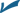Chapter 14

Matrices

A matrix is a rectangular array of numbers or other values, called elements. Matrices provide an excellent format for organizing information and performing computations involving that information.

Matrix arithmetic incorporates the operations of addition, subtraction, and multiplication, but in most unique ways. As with complex numbers, there is no division — the process is accomplished by multiplying by an inverse.

One of the first applications of matrices found in the classroom is that of solving systems of equations. It’s not usually easier to solve those systems with matrices than to do it with algebraic substitution and elimination, but the process is easily programmed in graphing calculators, making technology the choice for doing those systems problems.

The Problems You’ll Work On

In this chapter, you’ll work with matrices in the following ways:Determining matrix dimensionsAdding and subtracting matrices with the same dimensionPerforming scalar multiplicationMultiplying matrices with compatible dimensions

Finding the inverse of a square matrix

Dividing ...

Get 1001 Algebra II Practice Problems For Dummies now with the O’Reilly learning platform.

O’Reilly members experience books, live events, courses curated by job role, and more from O’Reilly and nearly 200 top publishers.